You can Download Samacheer Kalvi 6th Maths Book Solutions Guide Pdf, Tamilnadu State Board help you to revise the complete Syllabus and score more marks in your examinations.

## Tamilnadu Samacheer Kalvi 6th Maths Solutions Term 1 Chapter 1 Numbers Ex 1.6

Miscellaneous Practice ProblemsQuestion 1.
Try to open my locked suitcase which has the biggest 5 digits odd number as the password comprising the digits 7, 5, 4, 3 and 8. Find the password.
Solution:
Using a place value chart
The number should be the biggest odd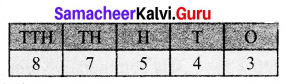The password is 87543

Question 2.
As per the census of 2001, the population of four states are given below. Arrange the states in ascending and descending order of their population.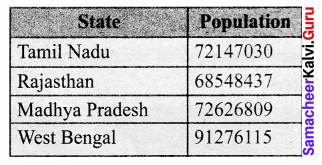Solution:
All the four values have 8 digits
Comparing the leftmost digits we have 91276115, 72626809, 72147030, 68548437
Descending order: 91276115 > 72626809 > 72147030 > 68548437
Ascending order: 68548437 < 72147030 < 72626809 < 91276115
Ascending order: Rajasthan < Tamil Nadu < Madhy Pradesh < West Bengal
Descending order: West Bengal > Madhya Pradesh > TamilNadu > Rajasthan

Question 3.
Study the following table and answer the questions.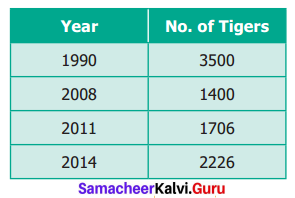(i) How many tigers were there in 2011?
(ii) How many tigers were less in 2008 than in 1990?
(iii) Did the number of tigers increase or decrease between 2011 and 2014? If yes, by how much?
Solution:
(i) There are 1706 tigers in 2011

(ii) No. of tigers in 2008 = 1400
No. of tigers in 1990 = 3500
There were 2100 lesser tigers

(iii) No. of tigers in 2014 = 2226
No. of tigers in 2011 = 1706
Difference = 520
2226 > 1706
The number of tigers increased from 2011 to 2014.
Yes, the number of tigers increased, 520 more tigers are there in 2014.Question 4.
Mullaikodi has 25 bags of apples. In each bag there are 9 apples. She shares them equally amongst her 6 friends. How many apples do each get? Are there any apples left over?
Solution:
No of apple bags = 25
Apples in each bag = 9
Total no of apples = 25 × 9 = 225
Apples shared among her 6 friends = 225 ÷ 6
So, among her 6 friends, each of them get 37 apples and 3 apples are left over.

Question 5.
A Poultry has produced 15472 eggs and fits 30 eggs in a tray. How many trays do they need?
Solution:
Total eggs = 15472
No. of eggs in 1 tray = 30
No. of trays needed = 15472 ÷ 30 = 516
No. of trays needed = 516 [515 + 1 for remaining 22 eggs]

Challenging Problems (Text book Page No.36 & 37)

Question 6.
Read the table and answer the following questions.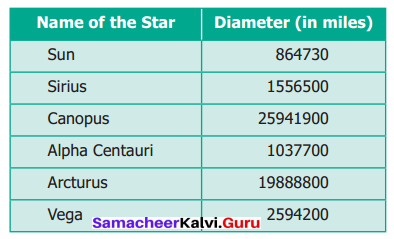Solution:
(i) Write the Canopus star’s diameter in words in the Indian and the International System.
(ii) Write the sum of the place values of 5 in Sirius star’s diameter in Indian System.
(iii) Eight hundred sixty-four million seven hundred thirty. Write in Indian System
(iv) Write the diameter in words of Arcturus star in the International System.
(v) Write the difference of the diameters of Canopus and Arcturus star in the Indian and the International Systems.
Solution:
(i) Canopus star’s diameter is 25941900 miles
Indian System: Two crores Fifty-Nine Lakh Forty-one thousand Nine Hundred
International System: Twenty-Five Million Nine Hundred Forty-One Thousand Nine Hundred.

(ii) Sirus star’s diameter = 1556500 miles
Sum of place values of 5 is 5 × 100000 + 5 × 10000 + 5 × 100
= 500000 + 50000 + 500
= 5,50,500

(iii) Given value is 864,000,730
In Indian System 86,40,00,730
Eighty-six crore forty lakhs seven hundred and thirty.

(iv) The diameter of the Arcturus Star is 19,888,800 miles
Nineteen Million Eight Hundred and Eighty-Eight Thousand Eight Hundred.

(v) The diameter of Canopus = 25941900
Diameter of Arcturus = 19888800
Difference = 6053100
In Indian System 60,53,100
Sixty lakh fifty-three thousand one hundred.
In International System 6,053,100
Six Million fifty-three thousand one hundred.Question 7.
Anbu asks Arivu Selvi to guess a five digit odd number. He gives the following hints.
i. The digit in the 1000s place is less than 5
ii. The digit in the 100s place is greater than 6
iii. The digit in the 10s place is 8
What is Arivu Selvi answer? Does she give more than one answer?
Solution:
63785, 53781

Question 8.
A Music concert is taking place in a stadium, A total of 7,689 chairs are to be put in rows of 90.
(i) How many rows will there be?
(ii) Will there be any chairs left over?
Solution:
(i) There will be 85 rows
(ii) Yes, there are 39 chairs left over.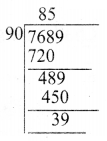Question 9.
Round off the seven-digit number 29,75,842 to the nearest lakhs and ten lakhs. Are they the same?
Solution: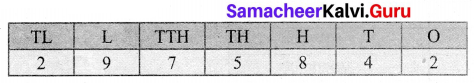To the nearest lakhs = 30,00,000
To the nearest ten lakhs = 30,00,000
Yes, they are the same.

Question 10.
Find the 5 or 6 or 7 digit numbers from a newspaper or a magazine to get a rounded number to the nearest ten thousand.
Solution:
(i) 14276 $$\simeq$$ 10000
(ii) 1,86945 $$\simeq$$ 1,90000